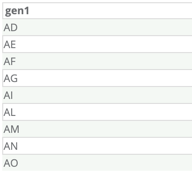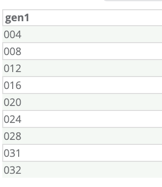CountryListGen -- Generates a list of countries in alphabetical or random order.

• dataToShow – this is required field. Determines what type of country data to show.  The choices are the following:
• twoCharacter
• threeCharacter
• numericCode
• fullName
• random – this is required field. Determines whether the countries will be generated in alphabetical order or in random order:
• if true then generates in random order
• If false then generates in alphabetical order
• Seed -  Defines an optional seed for randomization. Using the seed will ensure that the same random data is generated each time data is generated.Output:

• Fullname• twoCharacter• threeCharacter• numericCode